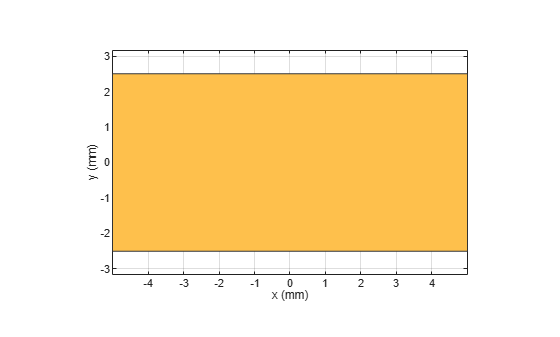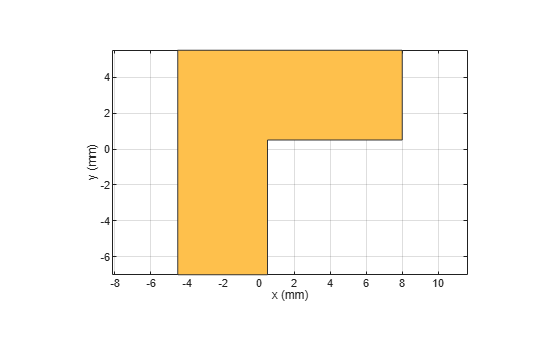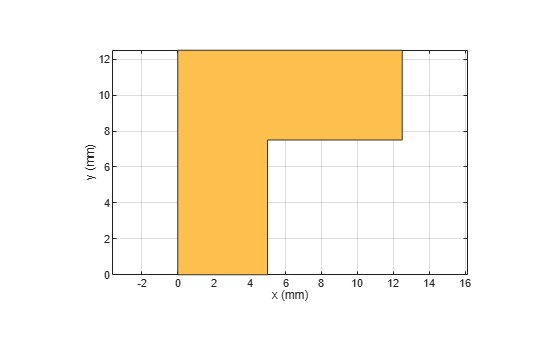Import and update Gerber files

Since R2021b

## Description

Use the `PCBReader` object to create a printed circuit board (PCB) reader to import Gerber files and to facilitate the creation of a PCB model. A Gerber file is a set of manufacturing files used to describe a PCB. A Gerber file uses an ASCII vector format to describe 2-D binary images.

## Creation

You can create a `PCBReader` object using the following methods:

• `gerberRead` — Create a `PCBReader` object with the specified Gerber and drill files.

• The `PCBReader` function described here.

### Syntax

``B = PCBReader(S)``
``B = PCBReader(Name=Value)``

### Description

example

````B = PCBReader(S)` creates a `PCBReader` object that imports multilayer PCB design files described in `S`. NoteTo translate the center of an imported symmetrical or asymmetrical polygon to `[0,0]`, please use one of the following MATLAB® functions: `boundingbox` and `centroid`. See examples, Translate Center of Imported Symmetrical Polygon to [0,0] and Translate Center of Imported Asymmetrical Polygon to [0,0].The `PCBReader` object reads RS-274X Gerber files. It does not support RS-274D Gerber files. ```
````B = PCBReader(Name=Value)` sets Properties using name-value arguments. `Name` is the property name and `Value` is the corresponding value. You can specify several name-value pair arguments in any order as Name1,Value1,...,NameN,ValueN. Properties not specified retain their default values. For example, ```B = PCBReader('StackUp',S,'Drillfile','ant.txt')``` imports the layer and drill files into the `PCBReader`.```

### Input Arguments

expand all

PCB stackup definition, specified as a `stackUp` object.

Example: `S = stackUp; B = PCBReader(S)`

Example: `B = PCBReader('StackUp',S)`

## Properties

expand all

PCB stackup definition, specified as a `stackUp` object.

Example: `S = stackUp; B.StackUp = S;`

Example: `B = PCBReader('StackUp',S)`

Name of Excellon drill file, specified as a character vector or string scalar. You can specify either a DRL or a TXT file.

Example: `B.DrillFile = 'ant.drl'`

Discretization points on curved segments, specified as a positive scalar.

Example: `B.NumPointsOnCurves = 80`

## Examples

collapse all

Create a PCB stack up definition object using default properties.

`S = stackUp;`

Set the thickness of the dielectric Air in layer 1 to 0.1 mm.

`S.Layer1.Thickness = 0.1e-3;`

Import a top layer Gerber file to layer 2.

`S.Layer2 = 'interdigital_Capacitor.gtl';`

Create a `PCBReader` object using the `stackUp` object, `S`.

`p = PCBReader('StackUp',S);`

To update the Gerber file, convert the `PCBReader` object to a `pcbComponent `object.

```pcbcapacitor = pcbComponent(p); pcbcapacitor.FeedDiameter = 0.001```
```pcbcapacitor = pcbComponent with properties: Name: 'interdigital_Capacitor' Revision: 'v1.0' BoardShape: [1x1 antenna.Rectangle] BoardThickness: 0.0061 Layers: {[1x1 dielectric] [1x1 antenna.Polygon] [1x1 dielectric] [1x1 dielectric]} FeedLocations: [0 0 2] FeedDiameter: 1.0000e-03 ViaLocations: [] ViaDiameter: [] FeedViaModel: 'square' Conductor: [1x1 metal] Tilt: 0 TiltAxis: [0 0 1] Load: [1x1 lumpedElement] ```

View the PCB component in the Gerber file.

`show(pcbcapacitor)`This example will show how to translate the symmetrical polygon imported from the Gerber file to the respective co-ordinates.

Create a PCB stackup and import rectangular patch on it.

```S = stackUp; S.Layer2 = 'PatchRectangular.gtl'; S.Layer3 = dielectric('Teflon');```

Use a PCB Reader to read the polygon shape from the stackup.

```p1 = PCBReader ('StackUp',S); figure; show(p1.shapes);```Translate the shape with center (0,0) using the `centriod` function from MATLAB.

`s = p1.shapes`
```s = Polygon with properties: Name: 'mypolygon' Vertices: [4x3 double] ```
```polygon = s; [x,y] = centroid(polygon); translate(polygon,[-x, -y, 0]);```This example shows how to translate the asymmetrical polygon imported from the Gerber file to the respective co-ordinates.

Create a PCB stackup and import rectangular patch on it.

```S = stackUp; S.Layer2 = 'RightAngleBend.gtl'; S.Layer3 = dielectric('Teflon');```

Use a PCB Reader to read the polygon shape from the stackup.

```p1 = PCBReader ('StackUp',S); figure; show(p1.shapes);```Translate the shape's bottom left corner to (0,0). Use the boundingbox function from MATLAB to convert the shape to polyshape and find the upper and lower bounds of the shape.

`s = p1.shapes`
```s = Polygon with properties: Name: 'mypolygon' Vertices: [6x3 double] ```
```ver = s.Vertices(:,1:2); polygon = polyshape(ver); [xlim, ylim] = boundingbox(polygon); translate(s,[-xlim(1), -ylim(1), 0]);```## Version History

Introduced in R2021b